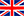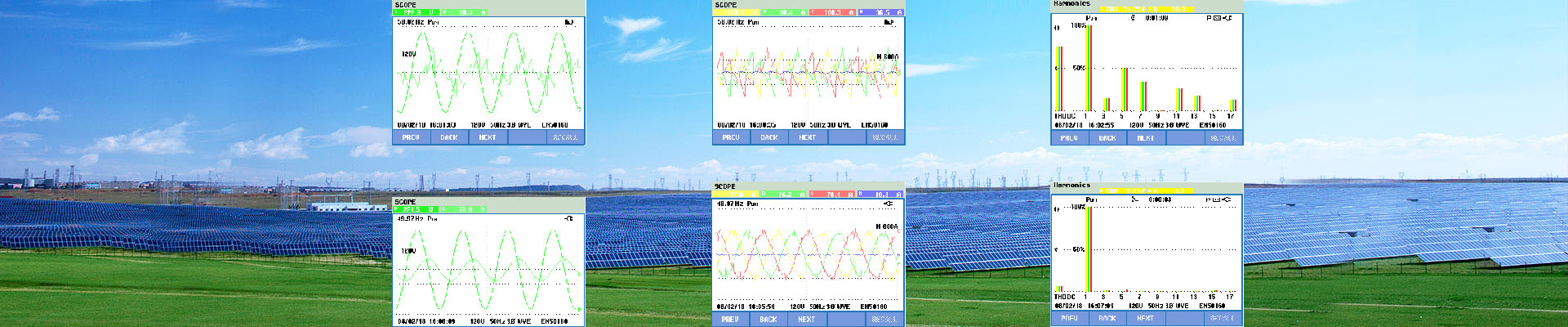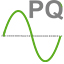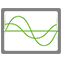English•#### Practical calculation of reactive power

Please refer to the following calculation examples Type of circuit Apparent power S (kVA) Active power P (kW) Reactive power Q (kVAr) Single phase (Ph + N) S = V x I P = V x I x cos φ Q = V x I x sin φ Single phase (Ph + Ph) S = U x I P = U x I x cos φ Q = U x I x sin φ Example:                                   5 kw load Cos φ= 0.5 10 kVA 5 Kw 8.7 kVAr Three-phase (3Ph or 3Ph+N) S = √3 x U x I P = √3 x U x I x cos φ Q = √3 x U x I x sin φ Example of Motor with               Pn = 51kW cos φ = 0.86                         efficiency = 0.91 65 kVA 56 kW 33 kVAr Calculations in the three-phase example were as follows: Pn = power supplied to the rotary axis = 51 kW P = active consumed power = Pn/ρ = 56 kW S = apparent power = P/cos φ = P/0.86 = 65 kVA Hence: Q = √(S² - P²) = √(65² - 56²) = 33 kVAr The average power factor values for various loads are given below.
view more
•#### STATCOM Applications

Electrical utilities and heavy industries face a number of challenges related to reactive power. Electrical utilities may be confronted with voltage sags, poor power factor and even voltage instability. Heavy industrial applications can cause disturbances like voltage unbalance, distortion or flicker on the electrical grid. Reactive power control can resolve these issues by improving the power factor or compensating for the voltage instability. In many cases, the traditional solutions of switching capacitors is too coarse and slow to stabilize a weak network. The most advanced solution to compensate reactive power is to incorporate a Voltage Source Converter (VSC) as a variable source of reactive power. These systems offer advantages compared to standard reactive power compensation solutions in demanding applications, such as wind farms and arc furnaces, where normal reactive power control generated by generators or capacitor banks alone are too slow for the sudden load changes. Typical STATCOM applications: –– Utilities with weak grids or fluctuating reactive loads –– Unbalanced loads –– Arc furnaces –– Wind farms –– Wood chippers –– Welding operations –– Car crushers & shredders –– Industrial mills –– Mining shovels, hoists and mills –– Harbor cranes
view more
•#### Dynamic Power Quality Technology for modern infrastructure

view more
•#### Active Filters

view more
•#### Sizing Active Harmonic Filter from Power Analyser Data

The Graph below shows Harmonic Current Distortion in the form of %THDi. This is hovering between 20 and 25%. Ideally, it should be less than 10% and preferably less than 8% THDi. The highest value of average total harmonic distortion (%THDi) across three phases was calculated from raw data. This value is 24.19%THDi and was recorded at time shown. At the same time, average line current (Amps) across three phases was 516.83A. Analysis of Findings From data above, Highest Average %THDi = 24.19% At the same time, Average I RMS across three phases was 516.83A. Taking X to be = fundamental frequency current I RMS = √(12 + 0.24192) * x = 516.83A 1.02884 * x = 516.83 x = 516.83 / 1.02884 = 502.34A Calculate harmonic current I RMS = √(502.342 + Harmonic Current2) = 516.83A 502.342 + Harmonic Current2 = 267113 Harmonic Current2 = 267113 – 252345 = 14767.8A Thus Harmonic current = 121.52A If %THDi = 8% (An appropriate value to satisfy electrical supply utilities) I RMS = √(502.342 + (0.08*502.34)2) = √(252345 + 1615) =503.94A I RMS = √(502.342 + Harmonic Current2) = 503.94A 502.342 + Harmonic Current2 = 253955.5 Harmonic Current2 = 253955.5 – 252345.5 = 1610A Thus Harmonic current = 40.125A Thus– To reduce % THDi at Incomer from 24.91% to 8% requires 81.4A of harmonic filtering (121.52-40.125). Active Harmonic filters come in sizes of 60A, 120A, 200A and 300A. To reduce %THDi to a level of 8% would require a 120A filter. Check performance when filter installed.
view more
•#### How to improve the power factor

Improving The power factor of an electrical installation consist of giving it the means to produce a varying proportion of the reactive energy that it consumes itself. Different systems are available to produce reactive energy, particularly phase advancers and shunt capacitors (or serial capacitors for major transport networks). The capacitor is most frequently used given: • It’s non‐consumption of active energy, • It’s purchasing cost, • It’s easy use, • It’s service life (approximately 10 year), • It’s very low maintenance (static device) The capacitor is a receiver composed of two conducting part (electrodes) separated by an insulator. When this receiver is subjected to a sinusoidal voltage, it shifts its current, and therefore it’s (capacitive reactive) power, by 90° forward the voltage. Conversely, all other receivers (motor, transformer, etc.) shift heir reactive component (inductive reactive power or current) 90° backward the voltage. The composition of these (inductive or capacitive) reactive powers or current gives a resulting reactive power or current below the existing value before the installation of Capacitors. In simpler terms, it can be said that inductive receivers (motors, transformers, etc.) cons energy, while capacitors (capacitive receivers) produce reactive energy.
view more
•#### Active Harmoni filter Applications

Many industrial facilities place poor power quality at the top of the list of inefficiency factors responsible for losses due to reduced productivity and lower quality of products. Optimal electrical power utilization becomes a challenge, as well as a necessity to keep up with everincreasing energy demand without drastic increases in energy costs. Large industrial, commercial and institutional power users can benefit from centralized medium voltage reactive power compensation systems. Medium voltage solutions typically require lower initial capital expenditures (\$/kVAR) than low voltage solutions while addressing most common power quality problems. Medium voltage metal-enclosed compensation systems provide centralized solution approach with attractive installation options supporting the scale and scope of large electrical services. Typical installations can be found at automotive, pulp/paper, steel, petrochemical, mining/mineral and other large industrial facilities. Many large commercial and institutional customers with medium voltage distribution network can also take advantage of medium voltage reactive compensation systems. Low voltage capacitor compensation systems can provide similar benefit of centralized solution at attractive costs for most mid and small industrial, commercial and institutional users. It offers very flexible, yet effective power factor compensation system in the low voltage network. An AHF can be used alone or in conjunction with other power quality correction equipment such as tuned harmonic filters, capacitor banks, etc. It can be placed in various locations within the electrical distribution network. Multiple units can be connected in parallel to provide higher compensation current to meet the TDD levels defined in IEEE519-1992 standard or levels defined in the plant operating requirements (5%-8%).
view more
•#### Reactive Power and Voltage Control of a Transmission Line

For understanding the relationship between the Reactive Power flow in a Transmission Line and Voltage drop, we will consider Short Transmission Line for simplicity. A short transmission line is one whose length is less than 80 km. For short Transmission Line Resistance and Reactance of line is assumed lumped. The important thing for short Transmission Line is that Shunt Capacitance is neglected because as the line is short the effect of shunt capacitance will be less while the reactance will predominate. By using the above philosophy we can represent a short Transmission Line as shown in figure below. Vs = Sending End Voltage Vr = Receiving End Voltage R = Line Resistance L = Line Inductance Z = Impedance of Line Is = Sending End Current Ir = Receiving End Current Now, The sending end Voltage Vs is related to the receiving end voltage Vr as below Vr ≈ Vs – ZIr where Z is the series impedance of the line consisting of resistance R and inductive reactance X. Z=R+jX Therefore, Vs – Vr ≈ ZIr ≈ RIrcosφ+ XIrsinφ ≈ (RP+XQ)/Vr as VrIrcosφ =P and VrIrsinφ =Q Now as R is quite small in comparison with X, it can be further simplified as: Vs – Vr ≈ (XQ)/Vr This expression indicates that following important points: The voltage drop for a given Receiving End Voltage Vr depends on Reactive Power Flow,Q. In a constant voltage line with Vs and Vr constant at all loads, then (XQ)/Vr is to be a constant which is achieved by varying Q as Vr tries to vary. Thus by controlling the Reactive Power flow through the Transmission Line voltage control is achieved.
view more
•#### STATCOM – Working Principle, Design and Application

What is SATACOM? STATCOM or Static Synchronous Compensator is a power electronic device using force commutated devices like IGBT, GTO etc. to control the reactive power flow through a power network and thereby increasing the stability of power network. STATCOM is a shunt device i.e. it is connected in shunt with the line. A Static Synchronous Compensator (STATCOM) is also known as a Static Synchronous Condenser (STATCON). It is a member of the Flexible AC Transmission System (FACTS) family of devices. The terms Synchronous in STATCOM mean that it can either absorb or generate reactive power in synchronization with the demand to stabilize the voltage of the power network. Working Principle of STATCOM: To understand the working principle of STATCOM, we will first have a look at the reactive power transfer equation. Let us consider two sources V1 and V2 are connected through an impedance Z = Ra + jX as shown in figure below. In the above reactive power flow equation, angle δ is the angle between V1 and V2. Thus if we maintain angle δ = 0 then Reactive power flow will become Q = (V2/X)[V1-V2] and active power flow will become P = V1V2Sinδ / X =0 To summarize, we can say that if the angle between V1 and V2 is zero, the flow of active power becomes zero and the flow of reactive power depends on (V1 – V2). Thus for flow of reactive power there are two possibilities. 1) If the magnitude of V1 is more than V2, then reactive power will flow from source V1 to V2. 2) If the magnitude of V2 is more than V1, reactive power will flow from source V2 to V1. This principle is used in STATCOM for reactive power control. Now we will discuss about the design of STATCOM for better correlation of working principle and design. Design of STATCOM: STATCOM has the following components: 1) A Voltage Source Converter, VSC The voltage-source converter is used to convert the DC input voltage to an AC output voltage. Two of the common VSC types are as below. a) Square-wave Inverters using Gate Turn-Off Thyristors: In this type of VSC, output AC voltage is controlled by changing the DC capacitor input voltage, as the fundamental component of the converter output voltage is proportional to the DC voltage. b) PWM Inverters using Insulated Gate Bipolar Transistors (IGBT): It uses Pulse Width Modulation (PWM) technique to create a sinusoidal waveform from a DC voltage source with a typical chopping frequency of a few kHz. In contrast to the GTO-based type, the IGBT-based VSC utilizes a fixed DC voltage and varies its output AC voltage by changing the modulation index of the PWM modulator. 2) DC Capacitor DC Capacitor is used to supply constant DC voltage to the voltage source converter, VSC. 3) Inductive Reactance A Transformer is connected between the output of VSC and Power System. Transformer basically acts as a coupling medium. In addition, Tranformer neutralize harmonics contained in the square waves produced by VSC. 4) Harmonic Filter Harmonic Filter attenuates the harmonics a...
view more
•#### Low voltage capacitor banks (TSC)

Introduction: Based on DSP control technology and Instantaneous power control theory, Wanlida thyristor switched capacitor banks (TSC/TSF) with high-speed switching capability are designed to support the supply voltage of distribution systems and to correct the power factor and eliminate the harmonic current of connected loads. Thyristor zero-crossing switching switches are resistant to mechanical wear, operate without noise, and are capable of practically transient-free switching, so it is more safety than contactor switched capacitor banks. Functions: 1.Improve power factor 2.Stabilize busbar voltage and suppress subsynchronous resonance 3.Eliminate harmonic current 4.Decrease grid loss 5.Optimize reactive power I6.ncrease transformer loading capacity Principles: Thyristor switched capacitor banks is the compensating device based on switching operation of capacitor banks by thyristor. TSC mainly consists of control system, thyristor, capacitors and reactors. Capacitor banks are divided into multiple units to realize step control. TSC is able to implement step regulating in reactive power, accuracy of regulating depends on numbers of multiple unit. Thyristor switched capacitor banks (TSC/TSF) apply delta wiring. To optimize effect of reactive power compensating and harmonic filtering, TSC capacity and branch configuration must be designed according to reactive power variation and harmonic components from nonlinear load. Technical features: SN Parameters Value 1 Rated voltage AC220V~1140V 2 Operation voltage 0.8~1.1Un 3 Rated frequency 50Hz/60Hz (Option) 4 Capacitor wiring mode Delta/Star (Option) 5 Switching mode Cycle/sequence/coded combination (Option) 6 Response time <20ms 7 Allowable maximum over current 1.3 times of rated current 8 Control steps 1~12/1~16 (Option) 9 Multiple units operation mode Parallel 10 Power loss <0.5% 11 Communication modes Modbus/RS485/232/CAN/GPRS etc (Option) 12 IP IP40 (customized) 13 Cooling mode Air cooling 14 Leading in mode Bottom/Top/Busbar (Option) 15 Altitude <1500 M 16 Ambient temperature -200C~+500C 17 Ambient humidity <95% 18 Operation environment No condensation, no corrupt gas, no conductive dust, no explosive and inflammable materials 19 Installation requirement No vibration, inclination≤50 20 Switching characteristic Current zero-crossing switching 21 Harmonic display Measure and display 2nd ~25th 22 Phase sequence protection Fault phase, phase loss 23 Thyristor protection Over-temperature protection and self-recovery 24 LCD display Real time current and voltage 25 Emergency protection Emergency stop switch 26 Compensation mode Three-phase compensation, phase splitting compensation 27 Protection modes Over current, over voltage, short-circuit, quick break
view more
•#### low voltage Capacitor banks (Contactors)

POWER FACTOR CORRECTION The power factor of a load is defined as the ratio of active power to apparent power, i.e. kW : kVA and is referred to as cos . The closer cos  is to unity, the less reactive power is drawn from the supply. For system with a low power factor the transmission of electric power in accordance with existing standards results in higher expenses both for the supply distribution companies and the consumer. In general terms, as the power factor of a three phase system decreases, the current rises. The heat dissipation in the system rises proportionately by a factor equivalent to the spare of the current. Types of Power Factor Correction : - Single or Fixed PFC, compensating for the reactive power of individual inductive loads at the point of connection so reducing the load in the connecting cables (typical for single, permanently operated loads with a constant power) - Group PFC, connecting on fixed capacitor to a group simultaneously operated inductive loads (e.g. group of motors, discharge lamp) - Bulk PFC, typical for large electrical systems with fluctuating load where it is common to connect a number of capacitors to 3 main power distribution station or substation. The capacitors are controlled by a microprocessor based relay which continuously monitors the reactive power demand on the supply. The relay connects or disconnects the capacitors to compensate for the actual reactive power of the total load and to reduce the overall demand of the supply.
view more
•#### Power Factor Correction

Devices consuming electrical energy present two types of loads: resistive and reactive. Those purely resistive are characterized because the current absorbed is placed in phase with the applied voltage. This is the case of incandescent lamps, electrical heaters, and so on.
view more
1 2

a total of 2 pages

welcome to zddq